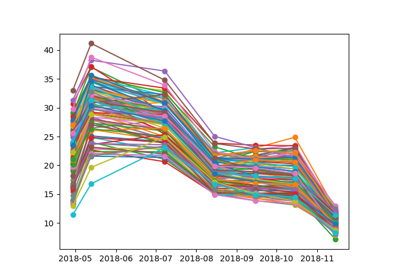# expression_manager¶

`museopheno.time_series.``expression_manager`(X, bands_order, expression, interpolate_nan=True, divide_X_by=1, multiply_by=1, order_by='date', dtype=<class 'numpy.float32'>)[source]

Generate expression/index from an array according to a bands_order, and expression.

The easiest way to use it is to choose a sensor from `museopheno.sensors`.

Parameters
• X (array.) – array where each line is a pixel.

• bands_order (list) – list of band order (e.g. [‘2’,’3’,’4’,’8’])

• expression (str or dict.) – If str, contains only the expression (e.g. ‘B8/B2’) If dict, contains a expression key and can contain a condition key. See museopheno.sensors.sensorManager.addIndice function.

• inteprolate_nan (boolean, default True, optional.) – If nan value a linear interpolation is done.

• divide_X_by (integer or float, default 1, optional.) – Value to divide X before computing the indice

• multiply_by (integer or float, default 1, optional.) – Value to multiply the result (e.g. 100 to set the NDVI between -100 and 100)

• order_by (str, default 'date', optional.) – if ‘date’, means your raster is stacked in this way : B1, B2 to Bx for the first date, then B1,B2 to Bx for the second date… if ‘band’, means your raster is stacked in this way : B1 first date, B1 second date… to B1 last date, then B2 first date…

• dtype (numpy dtype, default np.float32, optional.) – dtype of the output (e.g. np.int16 to store the NDVI in integer value)

Example

```>>> from museopheno import datasets, expressionManager
>>> X = datasets.Sentinel2_3a_2018(get_only_sample=True)
>>> indices.generateIndice(X,bands_order=['2','3','4','8','5','6','7','8A','11','12'],expression='B4/B8')
```

## Examples using `museopheno.time_series.expression_manager`¶